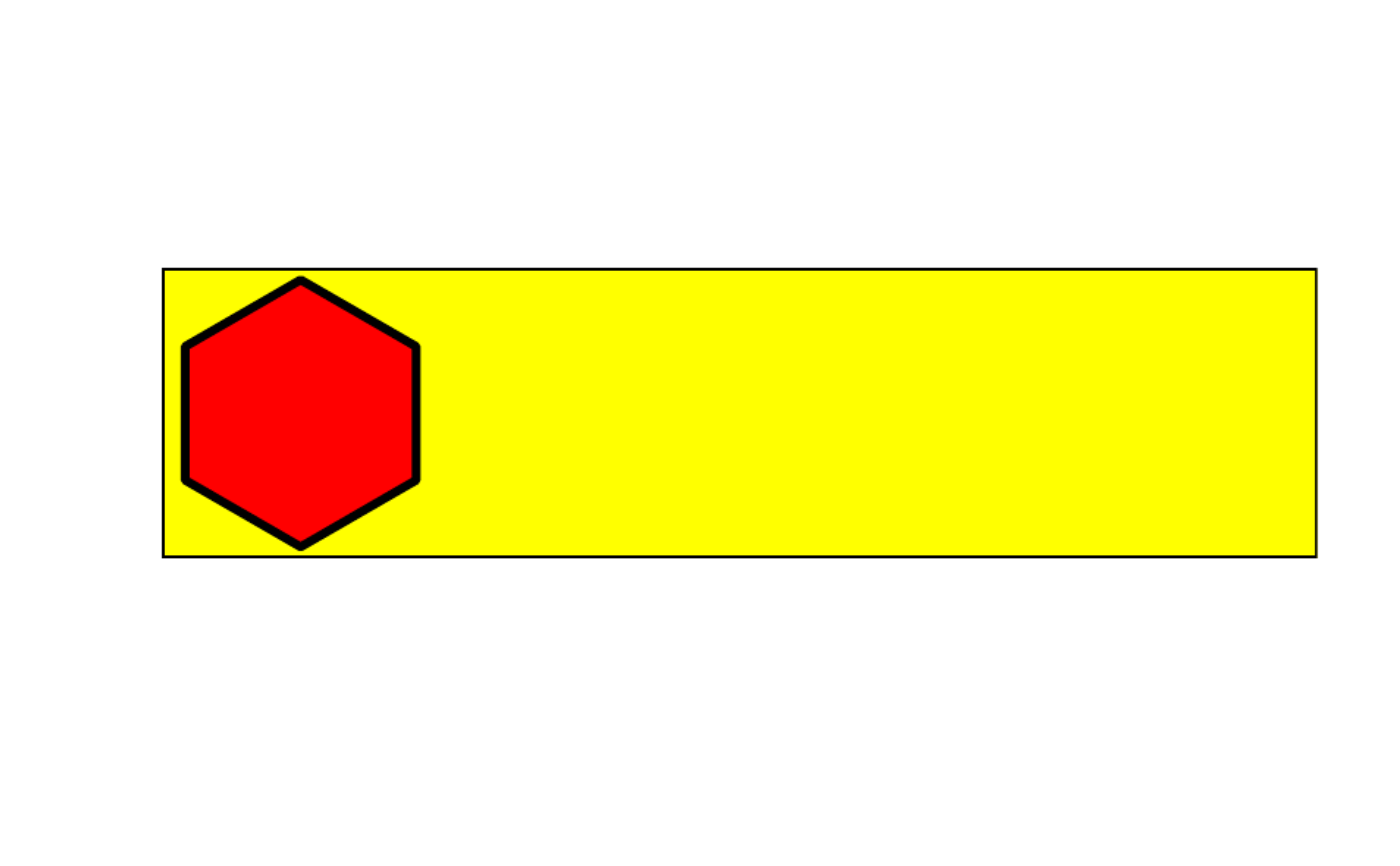Insert shape in label

## Usage

include_shape(
label,
value = "hexagon",
size = 5.08,
position = NA,
border_color = "black",
border_width = 1,
background = NA,
units = "cm",
panel_color = NA,
panel_size = NA
)

## Arguments

label

label output (table)

value

type of shape (string: "hexagon")

size

shape size (numeric: 5.08)

position

position coordinate (numeric: NA)

border_color

image color (string: "black")

border_width

shape line width (numeric: 1)

background

background color (string: "red")

units

units for shape (string: "cm")

panel_color

panel color (string: NA)

panel_size

panel size (numeric: NA)

data frame

## Examples


library(huito)

label <- label_layout(data = NA
, size = c(10, 2.5)
, background = "yellow"
) %>%
include_shape(
value = "hexagon"
, position = c(1.2, 1.25)
, background = "red"
, border_width = 1
, size = 2.4
#, panel_size = 2.4*1.157175
)

label %>% label_print("sample")ts <- label\$opts